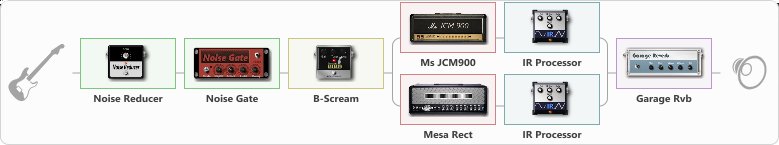# Dist.Carlos

Discussion in 'ToneLib-GFX presets' started by carlinos3, May 10, 2020.

1. Dist.Carlos

Preset name: Big Ben

Effects chain:Effect: "Noise Reducer" (Dynamics / Filter), active - "yes"
{
"Sens" = 51
"Mode" = Hard
}

Effect: "Noise Gate" (Dynamics / Filter), active - "yes"
{
"Mode" = Auto
"Depth" = 50
"Threshold" = 50
"Attack" = 0
"Hold" = 5
"Decay" = 250
}

Effect: "B-Scream" (Overdrive / Distortion), active - "yes"
{
"Drive" = 100
"Tone" = 26
"Level" = 76
}

Effect: "Splitter" (Dynamics / Filter), active - "yes"
{
"A-Bypass" = Off
"A-Pan" = -100
"A-Level" = 55
"B-Bypass" = Off
"B-Pan" = 100
"B-Level" = 55

'A' branch:
{

Effect: "Ms JCM900" (Amp simulators), active - "yes"
{
"Gain" = 100
"Bass" = 50
"Middle" = 51
"Treble" = 50
"Presence" = 68
"Master" = 60
"Level (dB)" = 3
}

Effect: "IR Processor" (Cabinets), active - "yes"
{
"IR" = charv.1_dc
"Low Cut (Hz)" = 0
"Hi Cut (kHz)" = 20.0
"Mix" = 100
"Level (dB)" = 0
}
}
'B' branch:
{

Effect: "Mesa Rect" (Amp simulators), active - "yes"
{
"Gain" = 100
"Bass" = 50
"Middle" = 41
"Treble" = 51
"Presence" = 78
"Master" = 65
"Level (dB)" = 3
}

Effect: "IR Processor" (Cabinets), active - "yes"
{
"IR" = charv.1_dc
"Low Cut (Hz)" = 0
"Hi Cut (kHz)" = 20.0
"Mix" = 100
"Level (dB)" = 6
}
}
}

Effect: "Garage Rvb" (Reverb), active - "yes"
{
"Time" = 5.2
"PreDelay" = 100
"LoDamp" = 0
"HiDamp" = 54
"Mix" = 42
}

Note: You will need to download and install the ToneLib-GFX software to use the preset.

#### Attached Files:

• ###### DistCarlos.tlgfx
File size:
117.7 KB
Views:
2,681
ktaxi and Niteha like this.